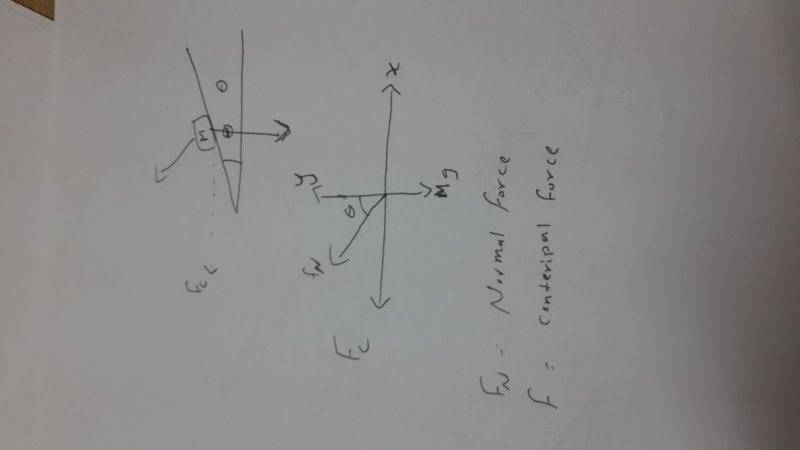# Bank curve problem

Is normal force on moving car on a track a component of weight or vice versa.

Because the centripetal force is aiming in the direction of x axis ,, The Normal Force would be resolved into to components one is equal to the weight of the moving object

It means that weight is component of normal force. Is centripetal force directed towards center of circle or towards the axis because these are two different cases.

If you draw a free body diagram of this problem what would you have ,,

the Mg lies on the negative y axis pointing toward the center of earth , the centripetal force is pointing toward either positive x axis or negative x axis [horizontal plane] , And the normal force would have an angel with the x-axis [which will be resolved into components]

If you draw a free body diagram of this problem what would you have ,,

the Mg lies on the negative y axis pointing toward the center of earth , the centripetal force is pointing toward either positive x axis or negative x axis [horizontal plane] , And the normal force would have an angel with the x-axis [which will be resolved into components]
I wanna ask that is centripetal force acting towards the center of circular track or towards its central axis(x direction)

To the center of the circle NOT to the center of the x-direction ,, I didn't mean that ,,

:D

To the center of the circle NOT to the center of the x-direction ,, I didn't mean that ,,

:D
If it is then the normal force is perpendicular to the track ( ie perpendicular to centripetal force) then it would have no component providing the requisite force. Here is the figure in photo.

#### Attachments

•14203180279401100976962.jpg
33.2 KB · Views: 412
Actually what you have drawn is somehow mistaken , The centripetal force direction is a horizontal line from the moving object to the center of the circular track ,,
See this picture ,,Actually what you have drawn is somehow mistaken , The centripetal force direction is a horizontal line from the moving object to the center of the circular track ,,
See this picture ,,
View attachment 77201
That was I wanted to confirm
You had first said that it is towards center but if it is so then the normal force would be at right angle to centripetal force as I drew but the figure you drew confirms that it is towards the axis. I took direction of normal force as y- axis.

Okay ,, The picture implies my point And sorry for my English
(:

Okay ,, The picture implies my point And sorry for my English
:)
Thank you for helping me. We done it together.

•Maged Saeed